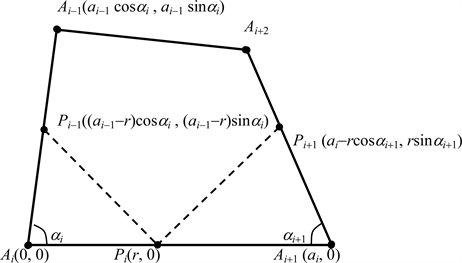# 正方形等截分之逆问题The Inverse Question of the Equal Segmentation Problem of Squares

DOI: 10.12677/PM.2019.93051, PDF, HTML, XML, 下载: 431  浏览: 1,777  国家自然科学基金支持

Abstract: In this paper, we focus on the inverse question of the equal segmentation problem of squares and obtain some interesting results.

1. 引言

$\underset{i=1}{\overset{4}{\sum }}{\alpha }_{i}=2\text{π}$ . (1)

2. 准备工作

$\left({a}_{i-1}-r\right)\mathrm{sin}{\alpha }_{i}+r\mathrm{cos}{\alpha }_{i+1}={a}_{i}-r$ ,(2)

$-\left({a}_{i-1}-r\right)cos{\alpha }_{i}+r\left(1-\mathrm{sin}{\alpha }_{i+1}\right)=0$ , (3)

${P}_{i}{P}_{i+1}=\left({a}_{i}-r-r\mathrm{cos}{\alpha }_{i+1},r\mathrm{sin}{\alpha }_{i+1}\right)$ ,

${P}_{i-1}{P}_{i}=\left(-\left({a}_{i-1}-r\right)\mathrm{cos}{\alpha }_{i}+r,-\left({a}_{i-1}-r\right)\mathrm{sin}{\alpha }_{i}\right)$ .

$\left(-\left({a}_{i}{}_{-1}-r\right)\mathrm{cos}{\alpha }_{i}+r-\left({a}_{i}{}_{-1}-r\right)\sqrt{-1}\mathrm{sin}{\alpha }_{i}\right)\sqrt{-1}={a}_{i}-r-r\mathrm{cos}{\alpha }_{i}{}_{+1}+r\sqrt{-1}\mathrm{sin}{\alpha }_{i}{}_{+1}$ , (4)Figure 1. Sketch map of equal segmentation

${a}_{i}-r-r\mathrm{cos}{\alpha }_{i+1}=\left({a}_{i-1}-r\right)\mathrm{sin}{\alpha }_{i}$ ,

$r\mathrm{sin}{\alpha }_{i+1}=-\left({a}_{i-1}-r\right)\mathrm{cos}{\alpha }_{i}+r$ .

$r\left(1+\mathrm{cos}{\alpha }_{i+1}-\mathrm{sin}{\alpha }_{i}\right)={a}_{i}-{a}_{i-1}\mathrm{sin}{\alpha }_{i}$ ,

$r\left(1+\mathrm{cos}{\alpha }_{i}-\mathrm{sin}{\alpha }_{i+1}\right)={a}_{i-1}\mathrm{cos}{\alpha }_{i}$ .

$1+\mathrm{cos}{\alpha }_{i+1}-\mathrm{sin}{\alpha }_{i}=0$ , ${a}_{i}-{a}_{i-1}\mathrm{sin}{\alpha }_{i}=0$ ,

$1+\mathrm{cos}{\alpha }_{i}-\mathrm{sin}{\alpha }_{i+1}=0$ , ${a}_{i-1}\mathrm{cos}{\alpha }_{i}=0$ ,

${\alpha }_{i}=\frac{\text{π}}{2}$ , ${a}_{i}={a}_{i-1}$ , $i=1,2,3,4$ .

$-\frac{{a}_{i-1}}{r}cos{\alpha }_{i}+cos{\alpha }_{i}+1-\mathrm{sin}{\alpha }_{i+1}=0$ , (5)

$\mathrm{sin}{\alpha }_{i-1}cos{\alpha }_{i}-\mathrm{sin}{\alpha }_{i}\mathrm{sin}\left({\alpha }_{i-1}+{\alpha }_{i}\right)+\mathrm{sin}{\alpha }_{i+1}cos{\alpha }_{i-1}=0$ , (6)

$\left({a}_{i-1}-r\right)\mathrm{sin}{\alpha }_{i}-\left({a}_{i}-r\right)+r\mathrm{cos}{\alpha }_{i+1}=0$ , (7)

$-\left({a}_{i}-r\right)cos{\alpha }_{i+1}+r\left(1-\mathrm{sin}{\alpha }_{i+2}\right)=0$ . (8)

$\begin{array}{c}\text{0}=|\begin{array}{ccc}\mathrm{sin}{\alpha }_{i}& -1& \mathrm{cos}{\alpha }_{i+1}\\ -\mathrm{cos}{\alpha }_{i}& 0& 1-\mathrm{sin}{\alpha }_{i+1}\\ 0& -\mathrm{cos}{\alpha }_{i+1}& 1-\mathrm{sin}{\alpha }_{i+2}\end{array}|\\ =|\begin{array}{cc}-\mathrm{cos}{\alpha }_{i}& 1-\mathrm{sin}{\alpha }_{i+1}\\ 0& 1-\mathrm{sin}{\alpha }_{i+2}\end{array}|+\mathrm{cos}{\alpha }_{i+1}|\begin{array}{cc}\mathrm{sin}{\alpha }_{i}& \mathrm{cos}{\alpha }_{i+1}\\ -\mathrm{cos}{\alpha }_{i}& 1-\mathrm{sin}{\alpha }_{i+1}\end{array}|\\ =-\mathrm{cos}{\alpha }_{i}\left(1-\mathrm{sin}{\alpha }_{i+2}\right)+\mathrm{cos}{\alpha }_{i+1}\left[\mathrm{sin}{\alpha }_{i}\left(1-\mathrm{sin}{\alpha }_{i+1}\right)+\mathrm{cos}{\alpha }_{i}\mathrm{cos}{\alpha }_{i+1}\right]\\ =-\mathrm{cos}{\alpha }_{i}\left(1-{\mathrm{cos}}^{2}{\alpha }_{i+1}\right)+\mathrm{cos}{\alpha }_{i}sin{\alpha }_{i+2}+\mathrm{cos}{\alpha }_{i+1}\mathrm{sin}{\alpha }_{i}-\mathrm{cos}{\alpha }_{i+1}\mathrm{sin}{\alpha }_{i}\mathrm{sin}{\alpha }_{i+1}\end{array}$

$\begin{array}{c}=\mathrm{cos}{\alpha }_{i}sin{\alpha }_{i+2}+\mathrm{cos}{\alpha }_{i+1}\mathrm{sin}{\alpha }_{i}-\left(\mathrm{cos}{\alpha }_{i}{\mathrm{sin}}^{2}{\alpha }_{i+1}+\mathrm{cos}{\alpha }_{i+1}\mathrm{sin}{\alpha }_{i}\mathrm{sin}{\alpha }_{i+1}\right)\\ =\mathrm{sin}{\alpha }_{i}\mathrm{cos}{\alpha }_{i+1}+\mathrm{cos}{\alpha }_{i}sin{\alpha }_{i+2}-\mathrm{sin}{\alpha }_{i+1}\left(\mathrm{cos}{\alpha }_{i}\mathrm{sin}{\alpha }_{i+1}+\mathrm{cos}{\alpha }_{i+1}\mathrm{sin}{\alpha }_{i}\right)\\ =\mathrm{sin}{\alpha }_{i}\mathrm{cos}{\alpha }_{i+1}+\mathrm{cos}{\alpha }_{i}sin{\alpha }_{i+2}-\mathrm{sin}{\alpha }_{i+1}\mathrm{sin}\left({\alpha }_{i}+{\alpha }_{i+1}\right)\end{array}$

$\left({a}_{j-1}-r\right)sin{\alpha }_{j}={a}_{j}-r$ , $\left({a}_{j-1}-r\right)\mathrm{cos}{\alpha }_{j}=0$ ,

${a}_{j}+r\mathrm{cos}{\alpha }_{j+2}={a}_{j+1}$ , $r\left(1-\mathrm{sin}{\alpha }_{j+2}\right)=0$ .

${\alpha }_{j+2}=\frac{\text{π}}{2}$ , ${\alpha }_{j}={a}_{j+1}$ .

$\left({a}_{j-1}-r\right)\mathrm{sin}{\alpha }_{j}={a}_{j}-r$ ,

$\left({a}_{j-1}-r\right)cos{\alpha }_{j}=0$ ,

$\mathrm{sin}{\alpha }_{j}>0$ , $\mathrm{cos}{\alpha }_{j}=0$ .

3. 主要结果

$\mathrm{sin}{\alpha }_{i-1}\mathrm{cos}{\alpha }_{i}-\mathrm{sin}{\alpha }_{i}\mathrm{sin}\left({\alpha }_{i-1}+{\alpha }_{i}\right)+\mathrm{sin}{\alpha }_{i+1}\mathrm{cos}{\alpha }_{i-1}=0$ , (9)

$\frac{{a}_{i-1}}{r}\text{\hspace{0.17em}}=1+\frac{1-\mathrm{sin}{\alpha }_{i+1}}{\mathrm{cos}{\alpha }_{i}}$ , (10)

$\left\{\begin{array}{l}{a}_{1}={c}_{0}\left(\mathrm{cos}{\alpha }_{1}+\mathrm{sin}{\alpha }_{1}-1\right)\\ {a}_{2}={c}_{0}\left(\mathrm{cos}{\alpha }_{1}-\mathrm{sin}{\alpha }_{1}+1\right)\\ r={c}_{0}\mathrm{cos}{\alpha }_{1}\end{array}$ , ${\alpha }_{1}\in \left(0,\frac{\text{π}}{\text{2}}\right)$ , ${c}_{0}\in \left(0,+\infty \right)$ . (11)

$\text{0}=\mathrm{sin}{\alpha }_{1}\mathrm{cos}{\alpha }_{\text{2}}+\mathrm{sin}{\alpha }_{\text{3}}\mathrm{cos}{\alpha }_{1}=\left(\mathrm{sin}{\alpha }_{\text{3}}-\mathrm{sin}{\alpha }_{1}\right)\mathrm{cos}{\alpha }_{1}$ ,

$\frac{{a}_{1}}{r}=1+\frac{1-\mathrm{sin}{\alpha }_{\text{3}}}{\mathrm{cos}{\alpha }_{\text{2}}}=1-\frac{1-\mathrm{sin}{\alpha }_{\text{1}}}{\mathrm{cos}{\alpha }_{\text{1}}}=\frac{\mathrm{cos}{\alpha }_{\text{1}}+\mathrm{sin}{\alpha }_{\text{1}}-1}{\mathrm{cos}{\alpha }_{\text{1}}}$ , (12)

$\frac{{a}_{2}}{r}=1+\frac{1-\mathrm{sin}{\alpha }_{\text{4}}}{\mathrm{cos}{\alpha }_{\text{3}}}=1+\frac{1-\mathrm{sin}{\alpha }_{\text{1}}}{\mathrm{cos}{\alpha }_{\text{1}}}=\frac{\mathrm{cos}{\alpha }_{\text{1}}-\mathrm{sin}{\alpha }_{\text{1}}+1}{\mathrm{cos}{\alpha }_{\text{1}}}$ , (13)

$\mathrm{sin}{\alpha }_{\text{1}}\mathrm{cos}{\alpha }_{\text{1}}-\mathrm{sin}{\alpha }_{\text{1}}\mathrm{sin}\left(\text{2}{\alpha }_{\text{1}}\right)+\mathrm{sin}{\alpha }_{\text{1}}\mathrm{cos}{\alpha }_{1}=0$ ,

$2\mathrm{sin}{\alpha }_{\text{1}}\mathrm{cos}{\alpha }_{\text{1}}\left[1-\mathrm{sin}{\alpha }_{\text{1}}\right]=0$ ,

$\frac{{a}_{1}}{{a}_{\text{2}}}=\frac{\mathrm{cos}{\alpha }_{\text{1}}-\mathrm{sin}{\alpha }_{\text{1}}+1}{\mathrm{cos}{\alpha }_{\text{1}}+\mathrm{sin}{\alpha }_{\text{1}}-1}=cot\frac{{\alpha }_{1}}{2}=\frac{\mathrm{sin}{\alpha }_{\text{1}}}{1-\mathrm{cos}{\alpha }_{\text{1}}}$ ,

$r=\frac{{a}_{1}+{a}_{\text{2}}}{2}$ .

$\underset{{\alpha }_{\text{1}}\to \frac{\text{π}}{2}}{\mathrm{lim}}\frac{{a}_{1}}{{a}_{\text{2}}}=1$ , $\underset{{\alpha }_{\text{1}}\to \frac{\text{π}}{2}}{\mathrm{lim}}\frac{{a}_{1}}{r}=1$ .

  张慧欣. 一个几何问题的思考[J]. 数学通报, 2012, 51(11): 53-54.  张新. 对正三角形等截分逆问题的再思考[J]. 数学通报, 2015, 54(5): 59-60.  王幼宁. 正三角形等截分逆问题的推广及其解析求解[J]. 数学通报, 2016, 55(5): 51-53.